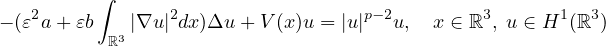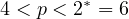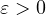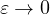Electron. J. Differential Equations, Vol. 2022 (2022), No. 57, pp. 1-23.

### Localized nodal solutions for semiclassical nonlinear Kirchhoff equations Lixia Wang

Abstract:
In this article, we consider the existence of localized sign-changing solutions for the semiclassical Kirchhoff equationwhere,is a small parameter, V(x) is a positive function that has a local minimum point P. When, by using a minimax characterization of higher dimensional symmetric linking structure via the symmetric mountain pass theorem, we obtain an infinite sequence of localized sign-changing solutions clustered at the point P.

Submitted April 18, 2022. Published August 2, 2022.
Math Subject Classifications: 35J20, 35J60.
Key Words: Kirchhoff equations; nodal solutions; penalization method.

Show me the PDF file (419 KB), TEX file for this article.Lixia Wang School of Sciences Tianjin Chengjian University Tianjin 300384, China email: wanglixia0311@126.com